﻿ 轴对称壳体结构声散射高效计算方法
 舰船科学技术2020, Vol. 42Issue (8): 55-59    DOI: 10.3404/j.issn.1672-7649.2020.08.010PDF

Research on efficient calculation method of acoustic scattering of axisymmetric shell structures
ZHOU Zi-xiang, REN Chun-yu
School of Naval Architecture and Ocean Engineering, Huazhong University of Science and Technology, Wuhan 430074, China
Abstract: Based on the variational theory of axisymmetric structures under non-axisymmetric excitations, a two-dimensional sound scattering characteristic analysis model of a rotating cylindrical shell structure is constructed in the finite element simulation software, which transforms three-dimensional spatial problems into two-dimensional planar problem and improves calculation efficiency. In this paper, the acoustic scattering of a capped cylindrical shell is first calculated and compared with the calculation results of the three-dimensional model in the literature. The results fit well, which illustrates the accuracy and applicability of the two-dimensional axisymmetric finite element method in this paper. Then, based on this method, the acoustic scattering characteristics of the underwater periodic stiffened cylindrical shell (including single shell and double shell structures) are studied, and the efficiency of the method is illustrated by comparing and analyzing the calculation efficiency with the three-dimensional models.
Key words: axisymmetric structure     finite element method     high efficiency
0 引　言

1 基本理论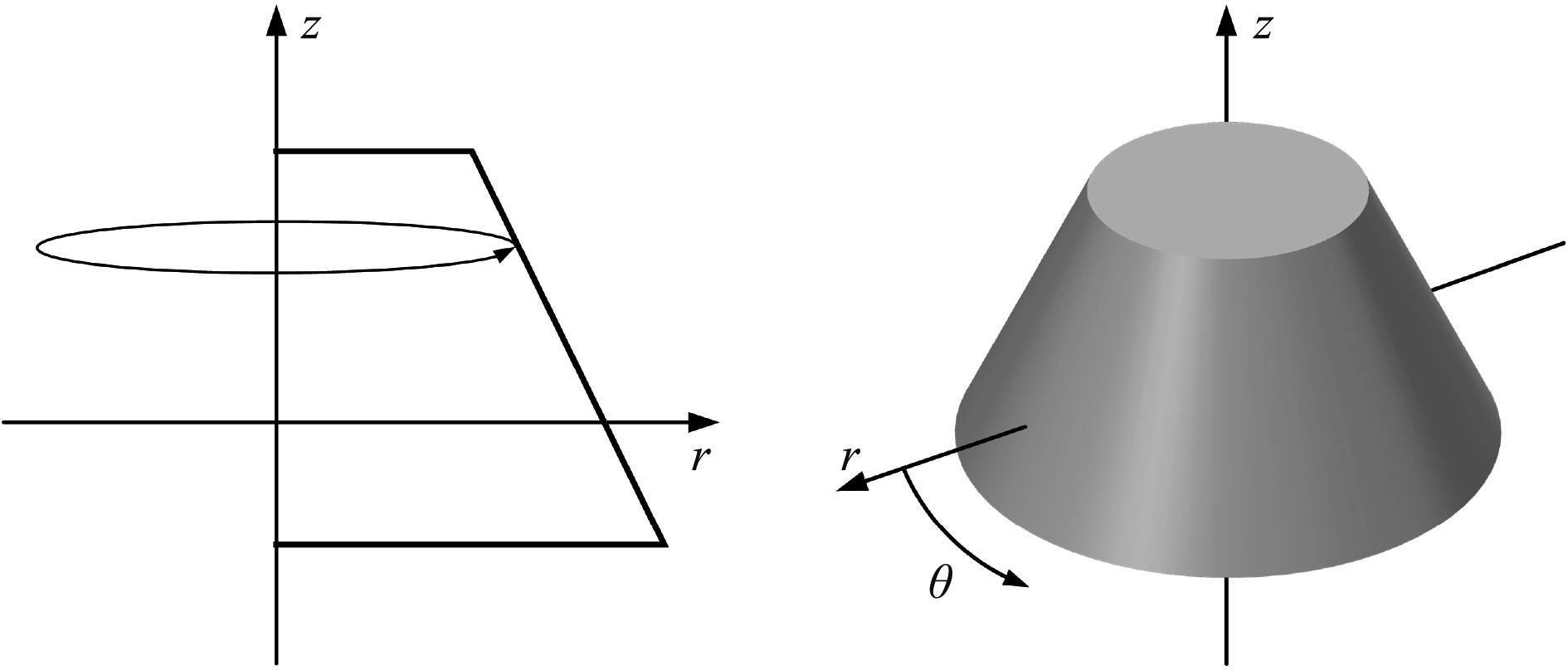图 1 旋转结构图 Fig. 1 Rotating structure
1.1 平面波展开

 ${p^{{\rm{inc}}}}(r,\theta ,z) = p_m^{{\rm{inc}}}(r,z){e^{im\theta }}, - \infty < m < + \infty {\text{，}}$ (1)

 $p_m^{{\rm{inc}}}(r,z) = {i^m}{e^{(ikz\sin \phi )}}{J_m}(kr\cos \phi ){\text{，}}$ (2)

1.2 声目标强度

 $TS({{r}}) = 20{\log _{10}}\frac{{{{r}}\left| {{p^{{\rm{scat }} ,\infty }}({{r}})} \right|}}{{\left| {{p^{{\rm{inc }}}}} \right|}}{\text{，}}$ (3)

1.3 非轴对称激励下轴对称目标的变分方程

 $\begin{split} & \int_{{\varOmega _s}} {\left[ {\begin{array}{*{20}{l}} { - {\omega ^2}{\rho _s}\left( {\left( {{u_m}\delta {u_m} + {w_m}\delta {w_m}} \right)\left\{ {\begin{array}{*{20}{l}} {2{\text π} } \\ {\text π} \end{array}} \right\} + {v_m}\delta {v_m}\left\{ {\begin{array}{*{20}{l}} 0 \\ {\text π} \end{array}} \right\}} \right) + } \\ {\left. {(\sigma _r^m\delta \left( {\varepsilon _r^m} \right) + \sigma _\theta ^m\delta \left( {\varepsilon _\theta ^m} \right) + \sigma _z^m\delta \left( {\varepsilon _z^m} \right)} \right)\left\{ {\begin{array}{*{20}{l}} {2{\text π} } \\ {\text π} \end{array}} \right\} + } \\ {\left( {2\sigma _{r\theta }^m\delta \left( {\varepsilon _{r\theta }^m} \right) + 2\sigma _{\theta z}^m\delta \left( {\varepsilon _{\theta z}^m} \right)} \right)\left\{ {\begin{array}{*{20}{l}} 0 \\ {\text π} \end{array}} \right\} + 2\sigma _{{r_2}}^m\delta \left( {\varepsilon _{rs}^m} \right) \times \left\{ {\begin{array}{*{20}{l}} {2{\text π} } \\ {\text π} \end{array}} \right\}} \end{array}} \right]} \times \hfill \\ & rdrdz + {I_{\partial {\Omega _s}}} = 0 \hfill {\text{，}}\\[-10pt] \end{split}$ (4)
 $\begin{split} \int_{{\varOmega _f}} & \left[ {\begin{array}{*{20}{l}} { - \frac{1}{{{\omega ^2}{\rho _f}}}\left( {\frac{{\partial p_m^{{{\rm scat}}}}}{{\partial r}}\delta \left( {\frac{{\partial p_m^{{{\rm scat}}}}}{{\partial r}}} \right)\left\{ {\begin{array}{*{20}{l}} {2{\text π} } \\ {\text π} \end{array}} \right\} + \frac{{{m^2}}}{{{r^2}}}p_m^{{{\rm scat}}}\delta \left( {p_m^{{{\rm scat}}}} \right) \times } \right.} \\ {\left. {\left\{ {\begin{array}{*{20}{l}} 0 \\ {\text π} \end{array}} \right\} + \frac{{\partial p_m^{{{\rm scat}}}}}{{\partial z}}\delta \left( {\frac{{\partial p_m^{{{\rm scat}}}}}{{\partial z}}} \right)\left\{ {\begin{array}{*{20}{l}} {2{\text π} } \\ {\text π} \end{array}} \right\}} \right) + \frac{1}{{{\rho _f}c_f^2}}p_m^{{{\rm scat}}}\delta \left( {p_m^{{{\rm scat}}}} \right)\left\{ {\begin{array}{*{20}{l}} {2{\text π} } \\ {\text π} \end{array}} \right\}} \end{array}} \right] \times \hfill \\ & r{{\rm d}}r{{\rm d}}z + {I_{\partial {\Omega _f}}} = 0 {\text{。}}\hfill \\[-10pt] \end{split}$ (5)

 ${{I_{\partial {\varOmega _s}}} = \int_{{\Gamma _{fsi}}} {\left( {p_m^{scat} + p_m^{inc}} \right)} \left( {{n_r}\delta {u_m} + {n_z}\delta {w_m}} \right)\left\{ {\begin{array}{*{20}{c}} {2{\text{π}} }\\ {\text{π}} \end{array}} \right\}r{\rm{d}}\ell }{\text{，}}$ (6)
 $\begin{split} & {I_{\delta {\varOmega _f}}} = \int_{{\Gamma _{fsi}}} {\left[ {{u_m}{n_r} + {u_z}{n_z} - \frac{1}{{{\omega ^2}{\rho _f}}}\left( {\frac{{\partial p_m^{inc}}}{{\partial r}}{n_r} + \frac{{\partial p_m^{inc}}}{{\partial z}}{n_z}} \right)} \right]} \times \hfill \\ & \quad \quad \quad \left\{ {\begin{array}{*{20}{l}} {2{\text π} } \\ {\text π} \end{array}} \right\}\delta p_m^{s{\text{cat}}}r{\rm{d}}\ell {\text{。}} \hfill \\[-20pt] \end{split}$ (7)

1.4 算例验证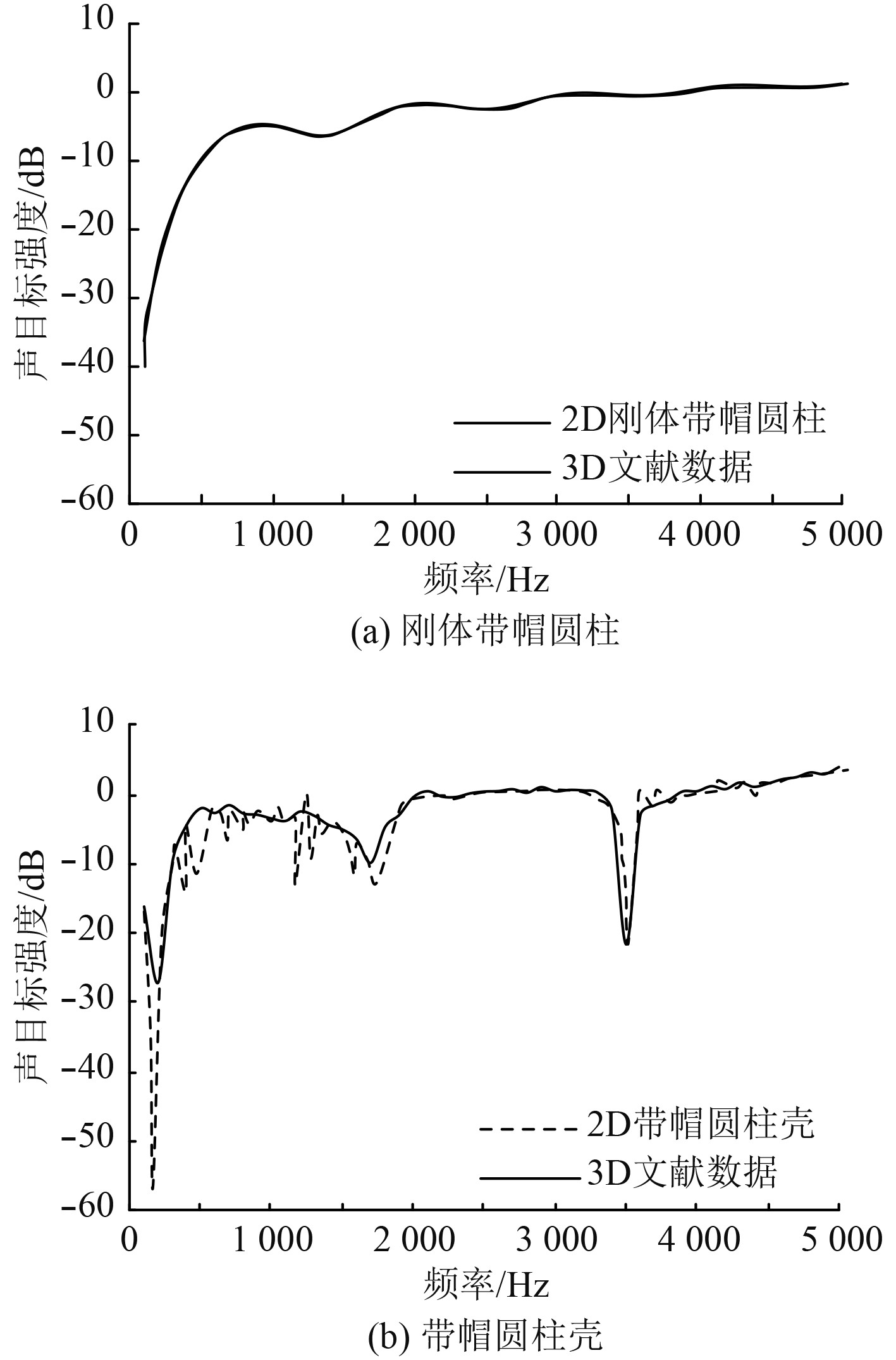图 2 声目标强度TS对比 Fig. 2 Comparison of acoustic target intensity TS
2 散射场计算与分析 2.1 目标模型与声学参数表 1 几何参数 Tab.1 Geometric parameters表 2 材料参数 Tab.2 Material parameters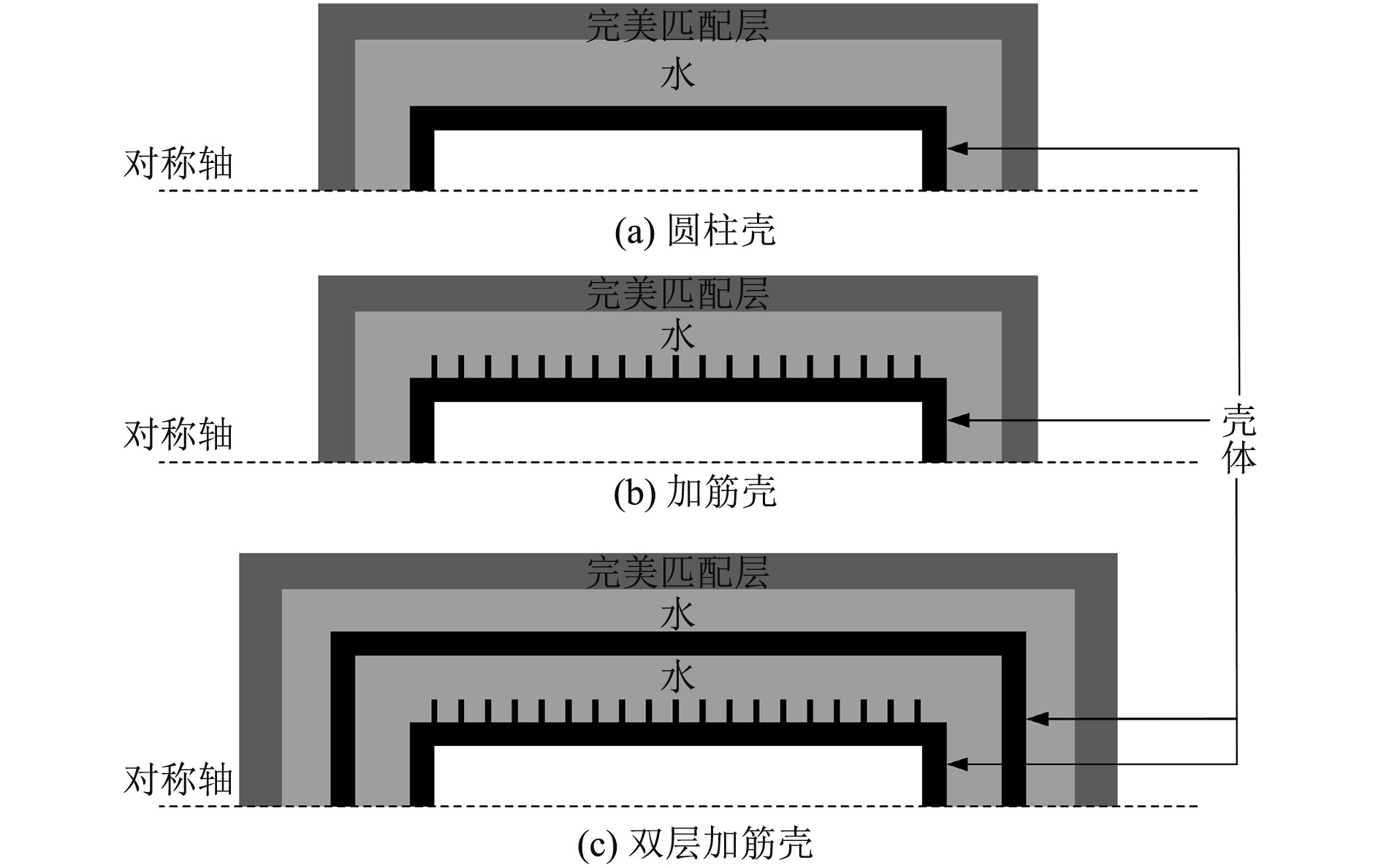图 3 二维轴对称模型 Fig. 3 Two-dimensional axisymmetric model
2.2 圆柱壳加筋对比分析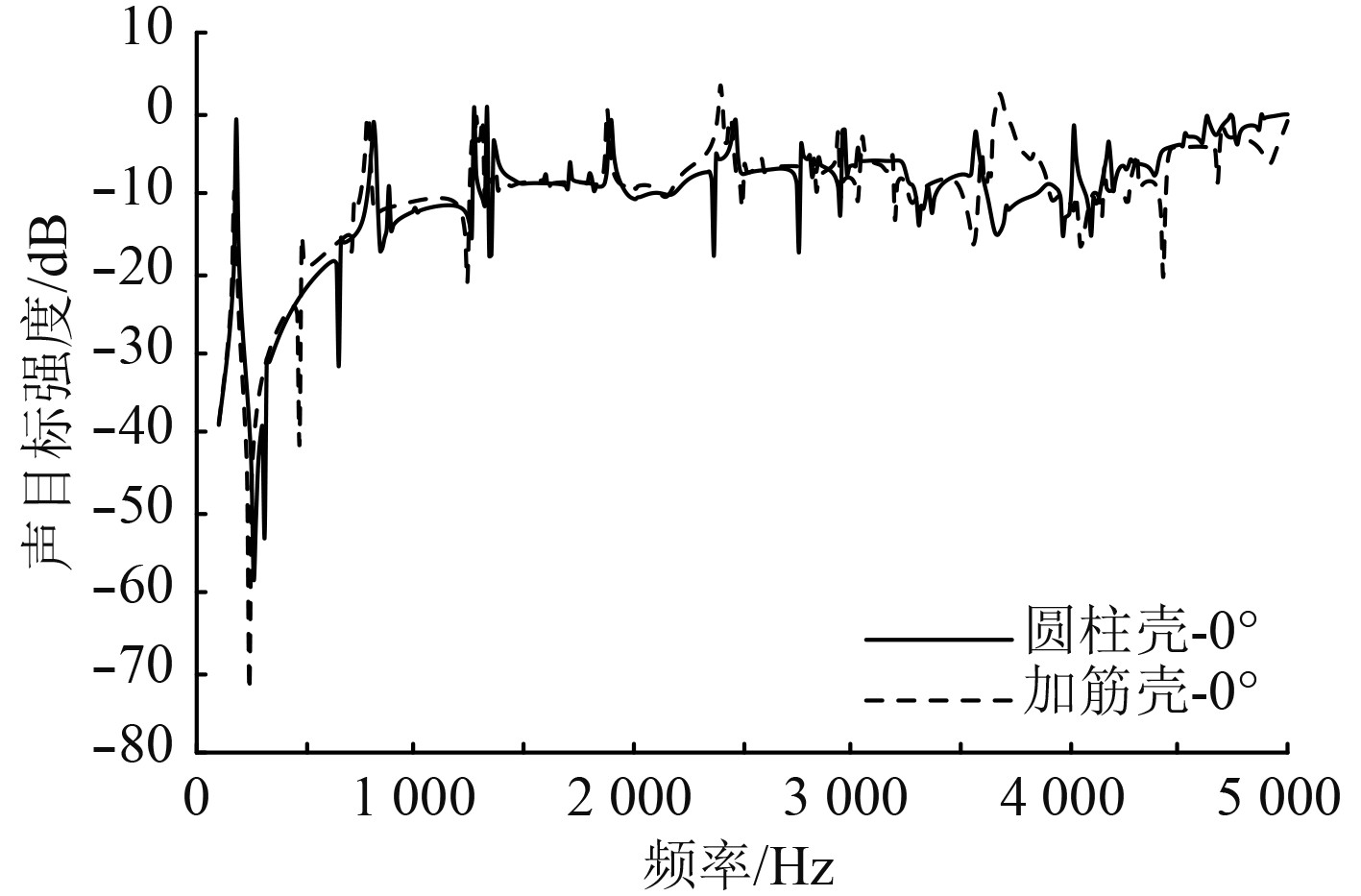图 4 圆柱壳与加筋壳声目标强度（0°） Fig. 4 Acoustic target intensity of cylindrical shell and stiffened shell（0°）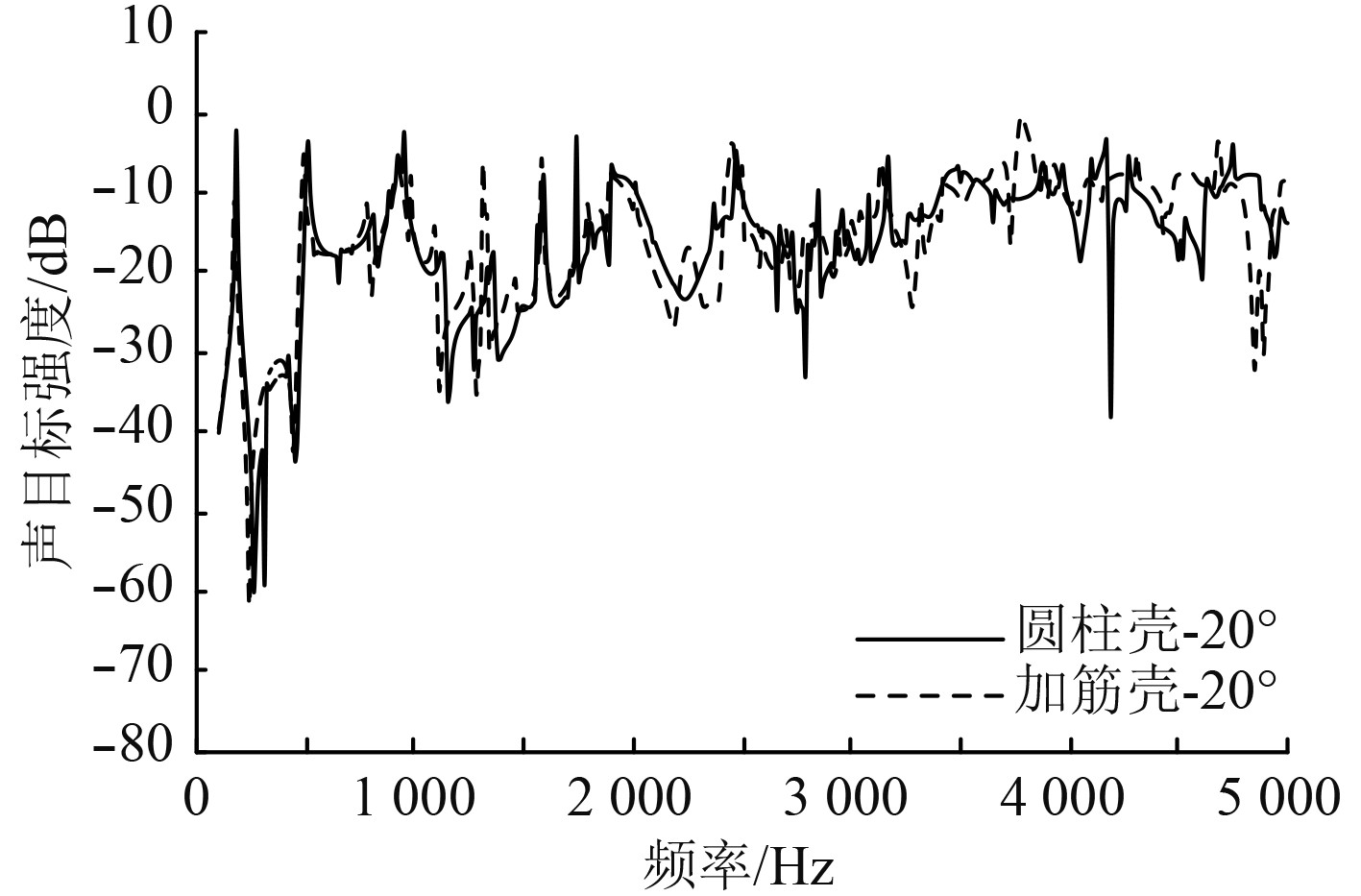图 5 圆柱壳与加筋壳声目标强度（20°） Fig. 5 Acoustic target intensity of cylindrical shell and stiffened shell（20°）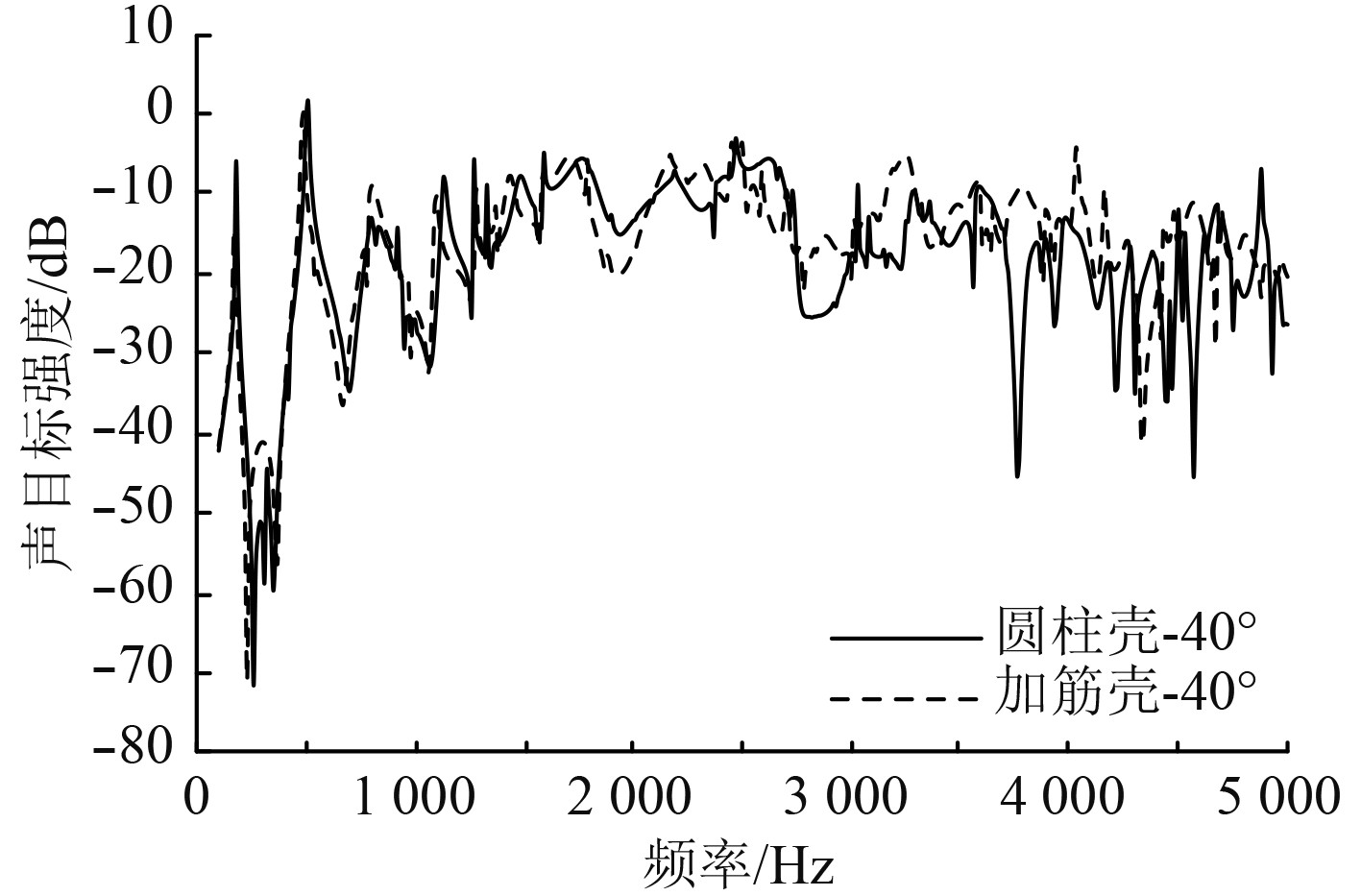图 6 圆柱壳与加筋壳声目标强度（40°） Fig. 6 Acoustic target intensity of cylindrical shell and stiffened shell（40°）

2.3 双层加筋圆柱壳对比分析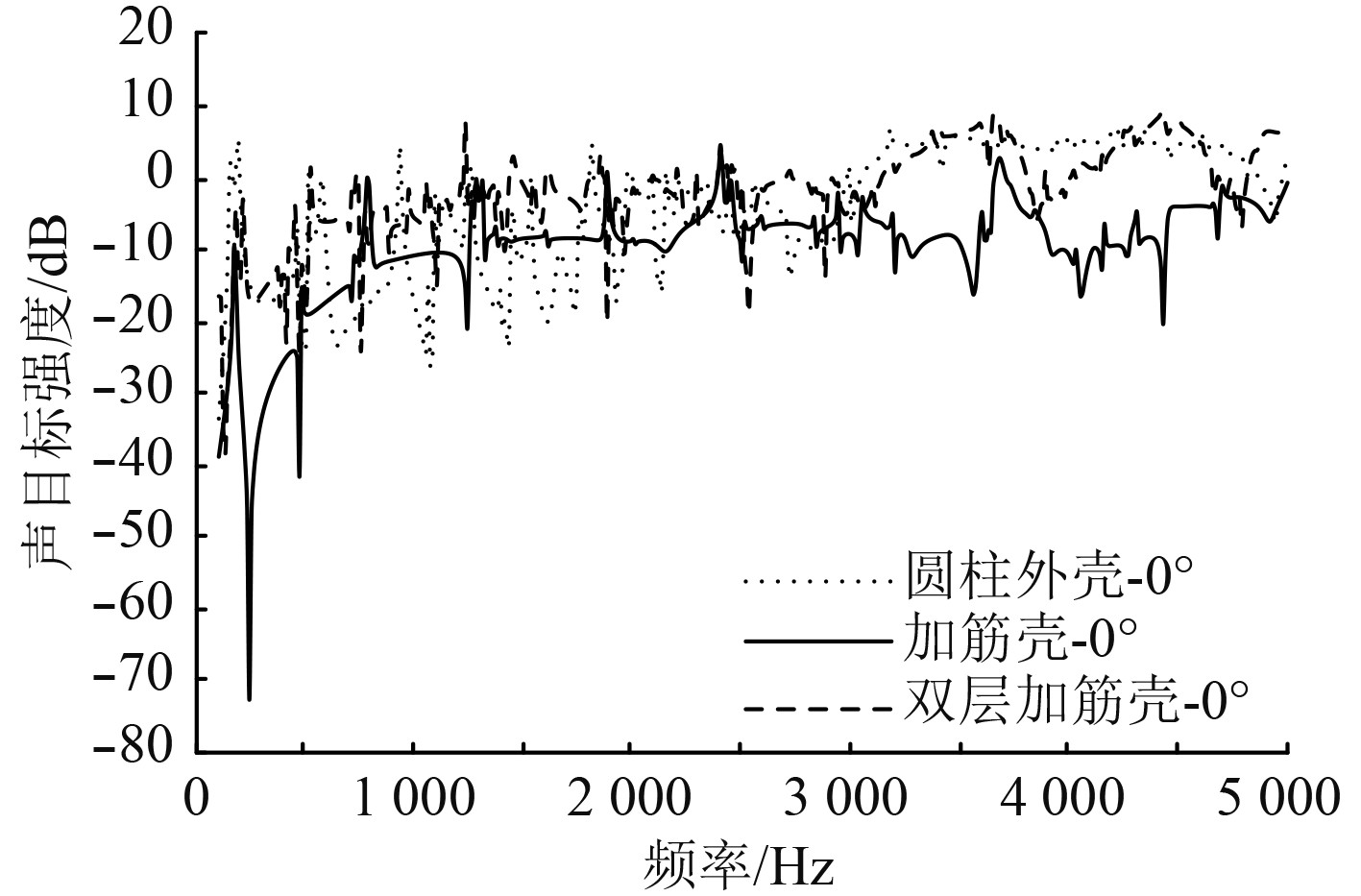图 7 加筋壳与双层加筋壳声目标强度（0°） Fig. 7 Acoustic target intensity of stiffened shell and double layer stiffened shell（0°）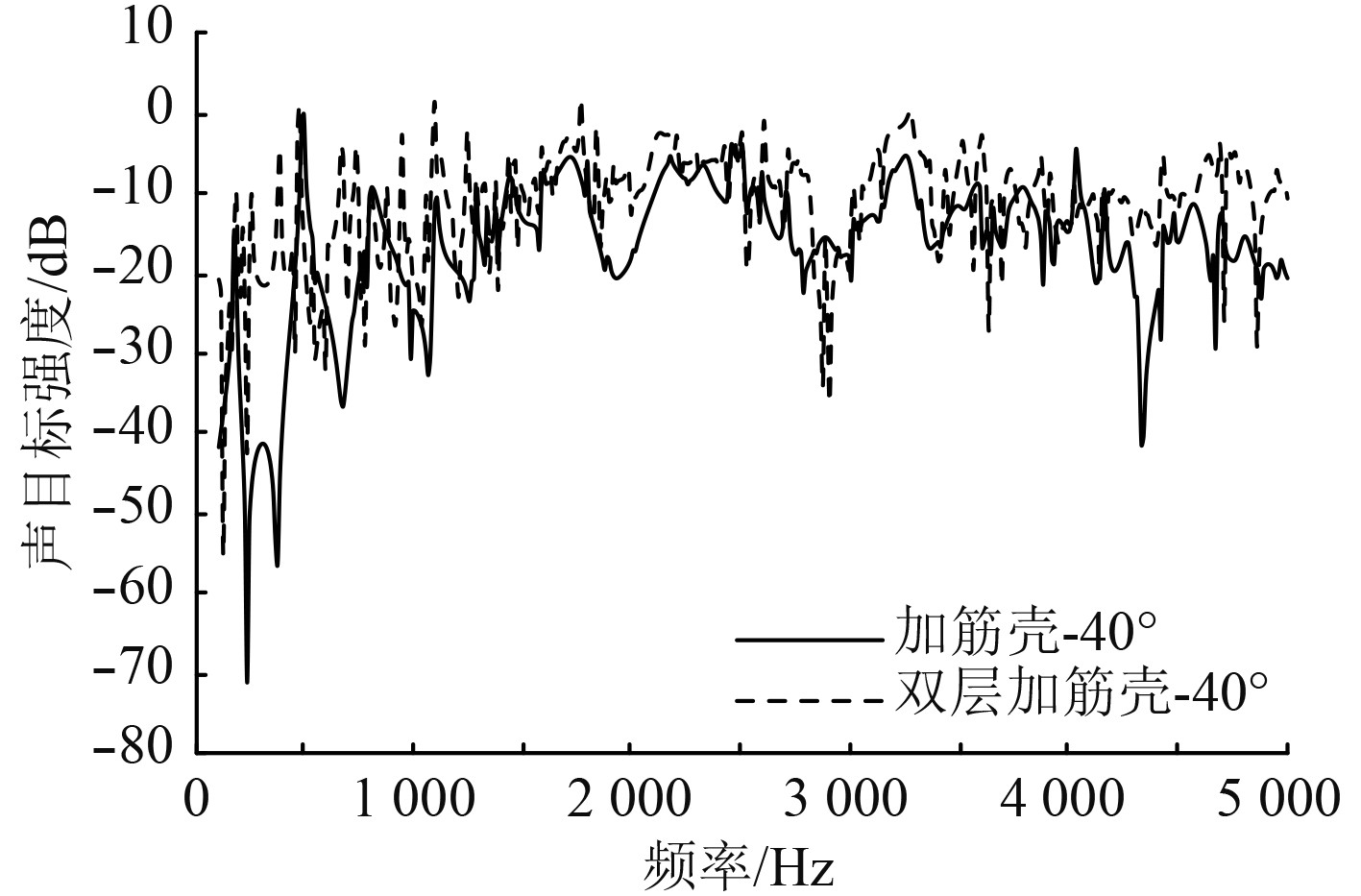图 9 加筋壳与双层加筋壳声目标强度（40°） Fig. 9 Acoustic target intensity of stiffened shell and double layer stiffened shell（40°）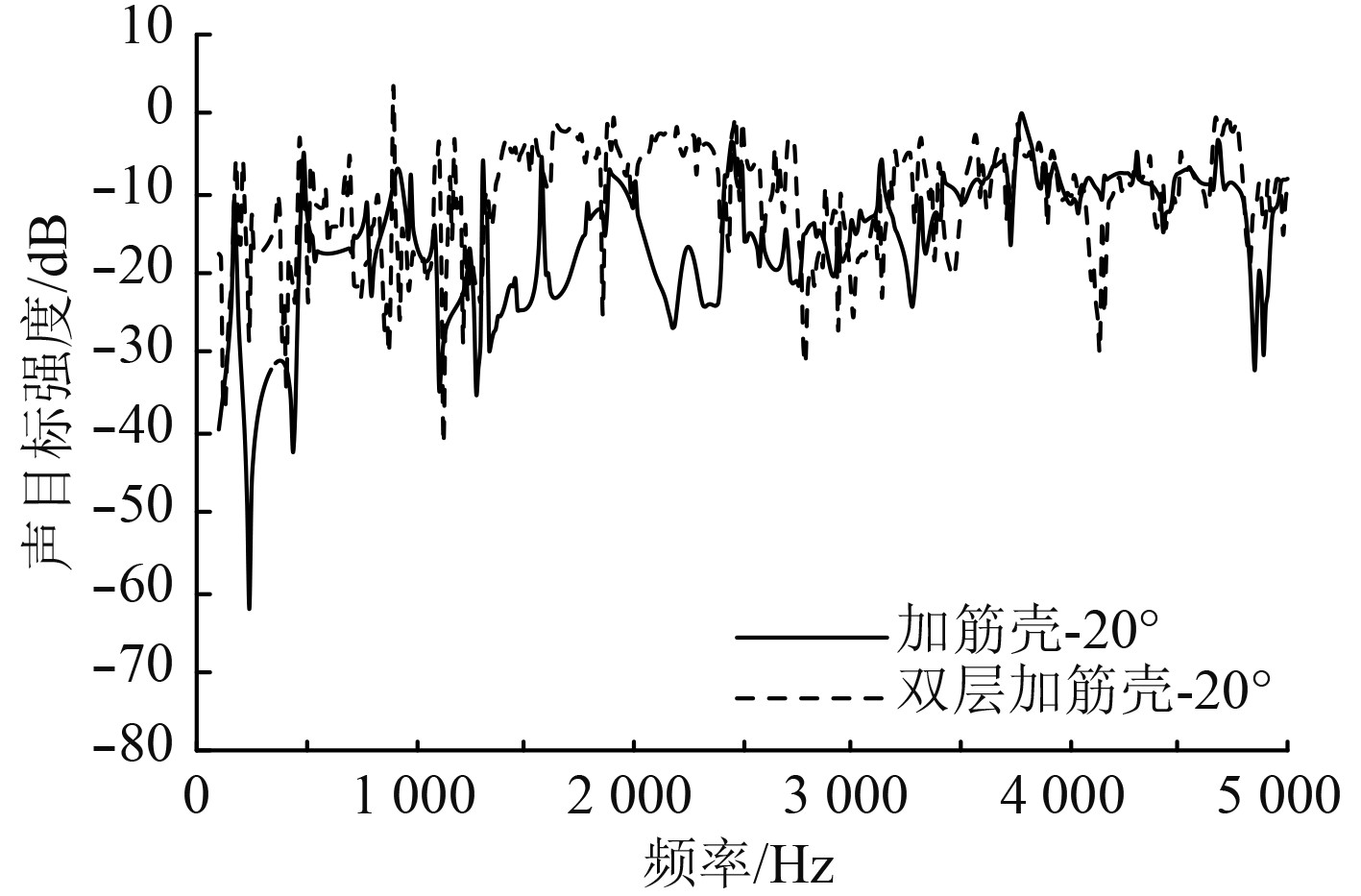图 8 加筋壳与双层加筋壳声目标强度（20°） Fig. 8 Acoustic target intensity of stiffened shell and double layer stiffened shell（20°）

2.4 计算效率对比分析表 3 各模型3D与2D计算效率对比 Tab.3 Comparison of 3D and 2D calculation efficiency of each model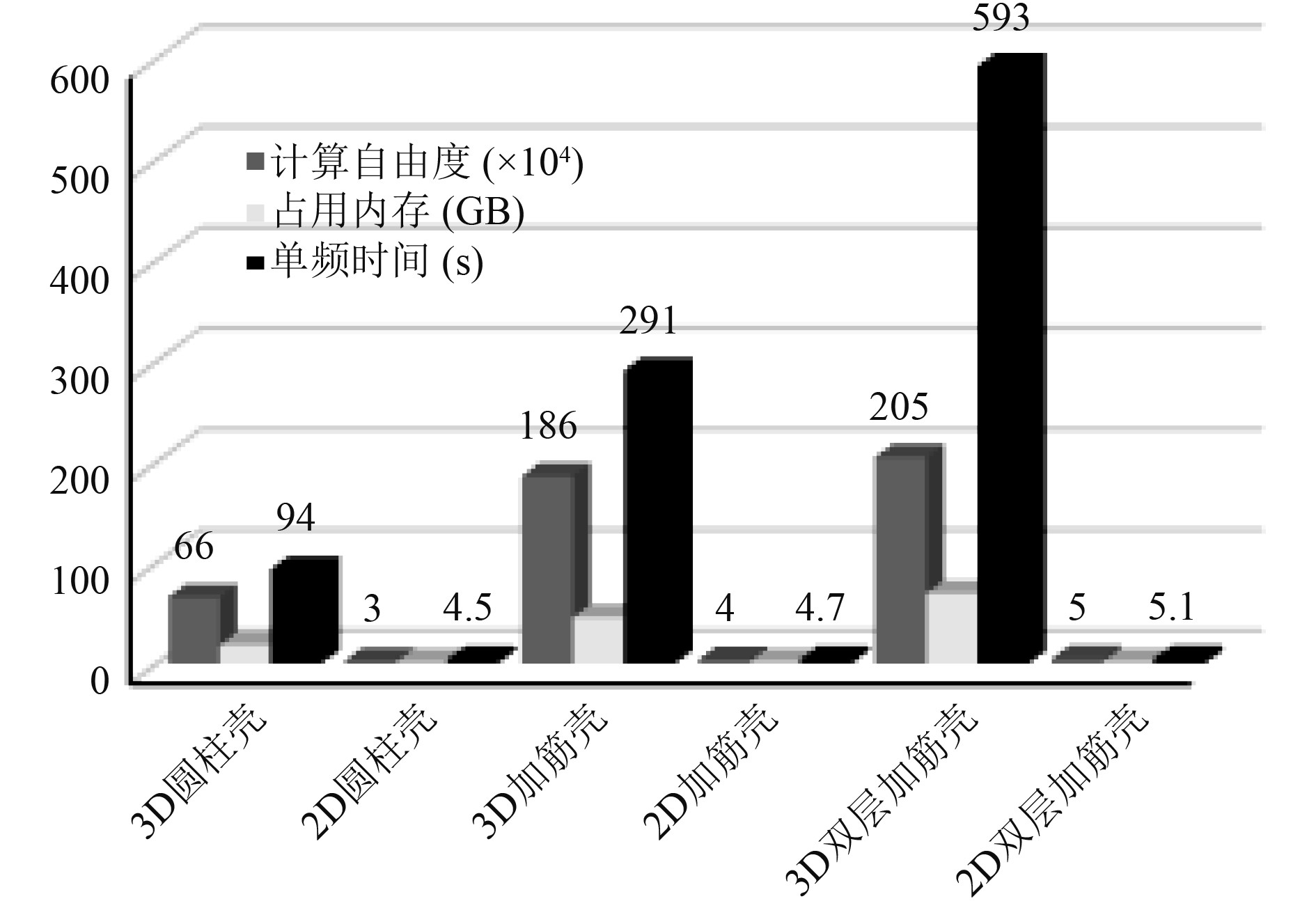图 10 各模型3D与2D计算效率对比 Fig. 10 Comparison of 3D and 2D calculation efficiency of each model

1）在最大网格尺寸不超过入射波波长1/6情况下，三维模型的计算自由度是二维模型的30～40倍；

2）二维模型运行内存占用基本稳定在2GB左右，三维模型运行内存占用随着结构复杂度升高，所需内存成倍增加；

3）计算时间上，二维模型平均单频点所需时间与三维模型相差20～100倍，远低于三维模型。如果考虑周向模数m，对于本文模型m=10已能满足计算精度，每个频点计算10次后，计算时间也与三维模型相差甚远。

3 结　语

  汤渭霖. 用物理声学方法计算非硬表面的声散射[J]. 声学学报, 1993(1): 45-53.  汤渭霖. 用物理声学方法计算界面附近目标的回波[J]. 声学学报, 1999(1): 1-5.  范威, 范军, 陈燕. 浅海波导中目标散射的简正波-Kirchhoff近似混合方法[J]. 声学学报, 2012(05): 13-21.  NUMRICH, S. K. Scattering of acoustic waves by a finite elastic cylinder immersed in water[J]. The Journal of the Acoustical Society of America, 1981, 70(5): 1407-1411. DOI:10.1121/1.387131  MACKOWSKI D W, MISHCHENKO M I. Calculation of the T matrix and the scattering matrix for ensembles of spheres[J]. Journal of the Optical Society of America A, 1996, 13(11): 2266-2278. DOI:10.1364/JOSAA.13.002266  WANG, Shuozhong. Finite-difference time-domain approach to underwater acoustic scattering problems[J]. The Journal of the Acoustical Society of America, 1996, 99(4): 1924-1931. DOI:10.1121/1.415375  TEZAUR R, MACEDO A, FARHAT C, et al. Three-dimensional finite element calculations in acoustic scattering using arbitrarily shaped convex artificial boundaries[J]. International Journal for Numerical Methods in Engineering, 2002, 53(6): 1461-1476. DOI:10.1002/nme.346  ISAKSON M J, CHOTIROS N P. Finite element modeling of acoustic scattering from fluid and elastic rough interfaces[J]. IEEE Journal of Oceanic Engineering, 2015, 40(2): 475-484. DOI:10.1109/JOE.2014.2313060  ESPANA A L, WILLIAMS K L, PLOTNICK D S, et al. Acoustic scattering from a water-filled cylindrical shell: Measurements, modeling, and interpretation[J]. The Journal of the Acoustical Society of America, 2014, 136(1): 109-121. DOI:10.1121/1.4881923  RAJABI M, AHMADIAN M T, JAMALI J. Acoustic scattering from submerged laminated composite cylindrical shells[J]. Composite Structures, 2015, 128: 395-405. DOI:10.1016/j.compstruct.2015.03.017  ZAMPOLLI M, TESEI A, JENSEN F B, et al. A computationally efficient finite element model with perfectly matched layers applied to scattering from axially symmetric objects[J]. The Journal of the Acoustical Society of America, 2007, 122(3): 1472-1485. DOI:10.1121/1.2764471  胡珍, 范军, 张培珍, 等. 水下掩埋目标的散射声场计算与实验[J]. 物理学报, 2015, 65(6).  张培珍, 李秀坤, 范军, 等. 局部固体填充的水中复杂目标声散射计算与实验[J]. 物理学报, 2016, 65(18): 273-281.